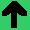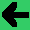# Lab 9: Linear Categoriser

1. Build a system that learns a linear classifier given data.
2. Make a new java project.
3. Put in an array of 2D points in two categories; the category can be represented by a third integer point.
4. Make a line that separates them. Remember, a line can be represented many ways including by two points, and by the equation x=my+b (where m is the slope constant and b is the offset from the origin).
5. In response to data, move the line. You can try the perceptron learning rule, or just move it gradually.
6. Try it with this data file.
7. Try it with this data file.
8. Can you make a plane for 3D?